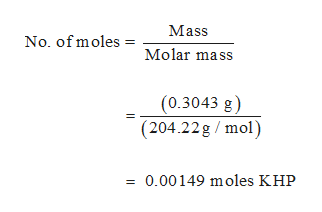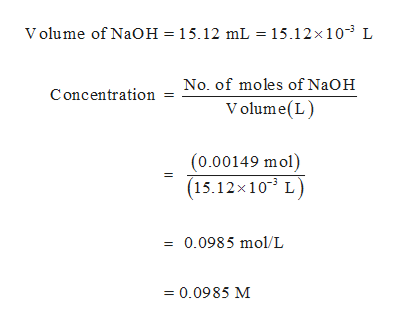# 0.3043 g of pure KHP was weighed out and titrated to an end point with 15.12 mL of a NaOH solution that was approximately 0.1 M. What is the exact concentration of the NaOH titrant?

Question
52 views

0.3043 g of pure KHP was weighed out and titrated to an end point with 15.12 mL of a NaOH solution that was approximately 0.1 M. What is the exact concentration of the NaOH titrant?

check_circle

Step 1

Neutralization reaction between KHP (C8H5KO4) and NaOH is given below,

Step 2

Number of moles of KHP reacted can be calculated as follows,help_outlineImage TranscriptioncloseMass No. of moles = Molar mass (0.3043 g) (204.22g mol) = 0.00149 moles KHP fullscreen
Step 3

From the balanced equation, it is clear that one moles of KHP reacts with one moles of NaOH. Hence, 0.00149 mo...help_outlineImage TranscriptioncloseVolume of NaOH = 15.12 mL = 15.12x 103 L No. of moles of NaOH Concentration Volume(L) (0.00149 mol) (15.12x 103 L) 0.0985 mol/L =0.0985 M fullscreen

### Want to see the full answer?

See Solution

#### Want to see this answer and more?

Solutions are written by subject experts who are available 24/7. Questions are typically answered within 1 hour.*

See Solution
*Response times may vary by subject and question.
Tagged in

### Quantitative analysis